# Making Straight Line Bends - Aircraft Sheet Metal Layout and Forming

When forming straight bends, the thickness of the material, its alloy composition, and its temper condition must be considered. Generally speaking, the thinner the material is, the more sharply it can be bent (the smaller the radius of bend), and the softer the material is, the sharper the bend is. Other factors that must be considered when making straight line bends are bend allowance, setback, and brake or sight line.

The radius of bend of a sheet of material is the radius of the bend as measured on the inside of the curved material. The minimum radius of bend of a sheet of material is the sharpest curve, or bend, to which the sheet can be bent without critically weakening the metal at the bend. If the radius of bend is too small, stresses and strains weaken the metal and may result in cracking.

A minimum radius of bend is specified for each type of aircraft sheet metal. The minimum bend radius is affected by the kind of material, thickness of the material, and temper condition of the material. Annealed sheet can be bent to a radius approximately equal to its thickness. Stainless steel and 2024-T3 aluminum alloy require a fairly large bend radius.

## Bending a U-Channel

To understand the process of making a sheet metal layout, the steps for determining the layout of a sample U-channel will be discussed. [Figure 1]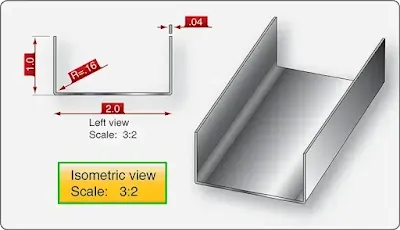Figure 1. U-channel bending example

When using bend allowance calculations, the following steps for finding the total developed length can be computed with formulas, charts, or computer-aided design (CAD) and computer-aided manufacturing (CAM) software packages. This channel is made of 0.040-inch 2024-T3 aluminum alloy.

### Step 1: Determine the Correct Bend Radius

Minimum bend radius charts are found in manufacturers’ maintenance manuals. A radius that is too sharp cracks the material during the bending process. Typically, the drawing indicates the radius to use, but it is a good practice to double check. For this layout example, use the minimum radius chart in Figure 2 to choose the correct bend radius for the alloy, temper, and the metal thickness. For 0.040, 2024-T3 the minimum allowable radius is 0.16-inch or 5⁄32-inch.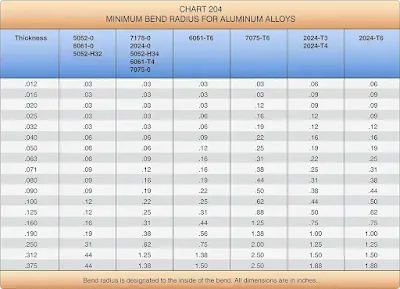Figure 2. Minimum bend radius (from the Raytheon Aircraft Structural Inspection and Repair Manual)

### Step 2: Find the Setback

The setback can be calculated with a formula or can be found in a setback chart available in aircraft maintenance manuals or Source, Maintenance, and Recoverability books (SMRs) [Figure 3]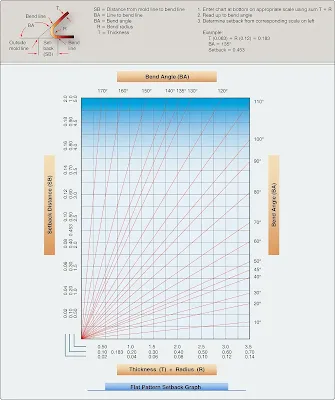Figure 3. Setback chart

Using a Formula to Calculate the Setback

SB = setback
K = K-factor (K is 1 for 90° bends)
R = inside radius of the bend
T = material thickness

Since all of the angles in this example are 90° angles, the setback is calculated as follows

SB = K(R+T) = 0.2 inches

NOTE: K = 1 for a 90° bend. For other than a 90° bend, use a K-factor chart.

#### Using a Setback Chart to Find the Setback

The setback chart is a quick way to find the setback and is useful for open and closed bends, because there is no need to calculate or find the K-factor. Several software packages and online calculators are available to calculate the setback. These programs are often used with CAD/CAM programs. [Figure 3]
• Enter chart at the bottom on the appropriate scale with the sum of the radius and material thickness.
• Read up to the bend angle.
• Find the setback from corresponding scale on the left.

Example:
• Material thickness is 0.063-inch.
• Bend angle is 135°.
• R + T = 0.183-inch.

Find 0.183 at the bottom of the graph. It is found in the middle scale.
• Read up to a bend angle of 135°.
• Locate the setback at the left hand side of the graph in the middle scale (0.435-inch). [Figure 3]

### Step 3: Find the Length of the Flat Line Dimension

The flat line dimension can be found using the formula:

Flat = MLD – SB
MLD = mold line dimension
SB = setback

The flats, or flat portions of the U-channel, are equal to the mold line dimension minus the setback for each of the sides, and the mold line length minus two setbacks for the center flat. Two setbacks need to be subtracted from the center flat because this flat has a bend on either side.

The flat dimension for the sample U-channel is calculated in the following manner:

Flat dimension = MLD – SB
Flat 1 = 1.00-inch – 0.2-inch = 0.8-inch
Flat 2 = 2.00-inch – (2 × 0.2-inch) = 1.6-inch
Flat 3 = 1.00-inch – 0.2-inch = 0.8-inch

### Step 4: Find the Bend Allowance

When making a bend or fold in a piece of metal, the bend allowance or length of material required for the bend must be calculated. Bend allowance depends on four factors: degree of bend, radius of the bend, thickness of the metal, and type of metal used.

The radius of the bend is generally proportional to the thickness of the material. Furthermore, the sharper the radius of bend, the less the material that is needed for the bend. The type of material is also important. If the material is soft, it can be bent very sharply; but if it is hard, the radius of bend is greater, and the bend allowance is greater. The degree of bend affects the overall length of the metal, whereas the thickness influences the radius of bend.

Bending a piece of metal compresses the material on the inside of the curve and stretches the material on the outside of the curve. However, at some distance between these two extremes lies a space which is not affected by either force. This is known as the neutral line or neutral axis and occurs at a distance approximately 0.445 times the metal thickness (0.445 × T) from the inside of the radius of the bend. [Figure 4]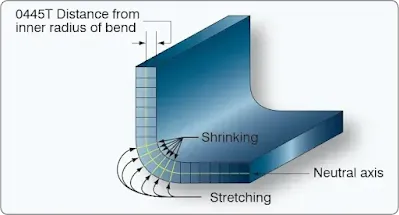Figure 4. Neutral axis and stresses resulting from bending

The length of this neutral axis must be determined so that sufficient material can be provided for the bend. This is called the bend allowance. This amount must be added to the overall length of the layout pattern to ensure adequate material for the bend. To save time in calculation of the bend allowance, formulas and charts for various angles, radii of bends, material thicknesses, and other factors have been developed.

Formula 1: Bend Allowance for a 90° Bend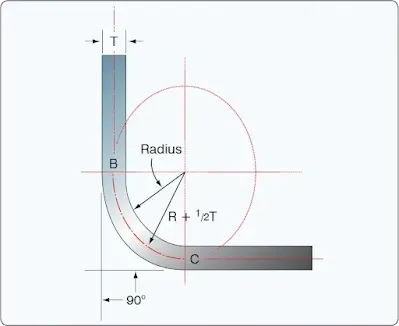Figure 5. Bend allowance for a 90° bend

To the radius of bend (R) add 1⁄2 the thickness of the metal (1⁄2T). This gives R + 1⁄2T, or the radius of the circle of the neutral axis. [Figure 5] Compute the circumference of this circle by multiplying the radius of the neutral line (R + 1⁄2T) by 2π (NOTE: π = 3.1416): 2π (R + 1⁄2T). Since a 90° bend is a quarter of the circle, divide the circumference by 4. This gives:

2π (R + 1⁄2T)
4

This is the bend allowance for a 90° bend. To use the formula for a 90° bend having a radius of 1⁄4 inch for material 0.051 inch thick, substitute in the formula as follows.The bend allowance, or the length of material required for the bend, is 0.4327 or 7⁄16-inch.

Formula 2: Bend Allowance for a 90° Bend
This formula uses two constant values that have evolved over a period of years as being the relationship of the degrees in the bend to the thickness of the metal when determining the bend allowance for a particular application. By experimentation with actual bends in metals, aircraft engineers have found that accurate bending results could be obtained by using the following formula for any degree of bend from 1° to 180°.

Bend allowance = (0.01743R + 0.0078T)N where:
R = the desired bend radius
T = the thickness of the metal
N = number of degrees of bend

To use this formula for a 90° bend having a radius of .16-inch for material 0.040-inch thick, substitute in the formula as follows:

Bend allowance = (0.01743 × 0.16) + (0.0078 × 0.040) × 90 = 0.27 inches

#### Use of Bend Allowance Chart for a 90° Bend

In Figure 6, the radius of bend is shown on the top line, and the metal thickness is shown on the left hand column. The upper number in each cell is the bend allowance for a 90° bend. The lower number in the cell is the bend allowance per 1° of bend. To determine the bend allowance for a 90° bend, simply use the top number in the chart.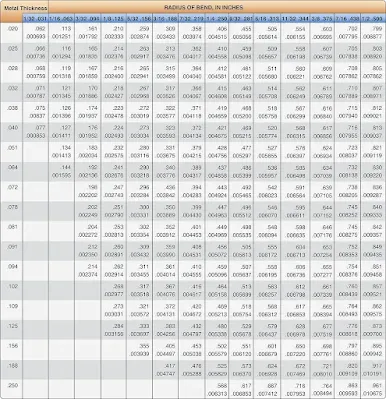Figure 6. Bend allowance

Example: The material thickness of the U-channel is 0.040-inch and the bend radius is 0.16-inch.

Reading across the top of the bend allowance chart, find the column for a radius of bend of .156-inch. Now, find the block in this column that is opposite the material thickness (gauge) of 0.040 in the column at the left. The upper number in the cell is (0.273), the correct bend allowance in inches for a 90° bends.

Several bend allowance calculation programs are available online. Just enter the material thickness, radius, and degree of bend and the computer program calculates the bend allowance.

#### Use of Chart for Other Than a 90° Bend

If the bend is to be other than 90°, use the lower number in the block (the bend allowance for 1°) and compute the bend allowance.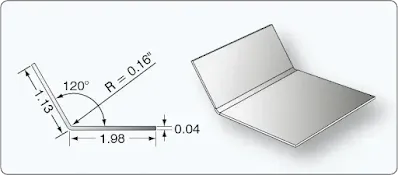Figure 7. Bend allowance for bends less than 90°

Example:
The L-bracket shown in Figure 7 is made from 2024-T3 aluminum alloy and the bend is 60° from flat. Note that the bend angle in the figure indicates 120°, but that is the number of degrees between the two flanges and not the bend angle from flat. To find the correct bend angle, use the following formula:

Bend Angle = 180° – Angle between flanges

The actual bend is 60°. To find the correct bend radius for a 60° bend of material 0.040-inches thick, use the following procedure.
1. Go to the left side of the table and find 0.040-inch.
2. Go to the right and locate the bend radius of 0.16-inch (0.156-inch).
3. Note the bottom number in the block (0.003034).
4. Multiply this number by the bend angle:0.003034 × 60 = 0.18204

### Step 5: Find the Total Developed Width of the Material

The total developed width (TDW) can be calculated when the dimensions of the flats and the bend allowance are found. The following formula is used to calculate TDW:

TDW = Flats + (bend allowance × number of bends)

For the U-channel example, this gives:
TDW = Flat 1 + Flat 2 + Flat 3 + (2 × BA)
TDW = 0.8 + 1.6 + 0.8 + (2 × 0.27)
TDW = 3.74-inches

Note that the amount of metal needed to make the channel is less than the dimensions of the outside of the channel (total of mold line dimensions is 4 inches). This is because the metal follows the radius of the bend rather than going from mold line to mold line. It is good practice to check that the calculated TDW is smaller than the total mold line dimensions. If the calculated TDW is larger than the mold line dimensions, the math was incorrect.

### Step 6: Flat Pattern Lay Out

After a flat pattern layout of all relevant information is made, the material can be cut to the correct size, and the bend tangent lines can be drawn on the material. [Figure 8]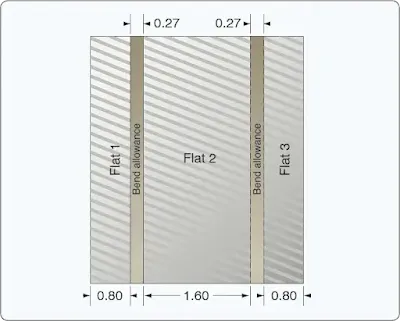Figure 8. Flat pattern layout

### Step 7: Draw the Sight Lines on the Flat Pattern

The pattern laid out in Figure 8 is complete, except for a sight line that needs to be drawn to help position the bend tangent line directly at the point where the bend should start. Draw a line inside the bend allowance area that is one bend radius away from the bend tangent line that is placed under the brake nose bar. Put the metal in the brake under the clamp and adjust the position of the metal until the sight line is directly below the edge of the radius bar. [Figure 9] Now, clamp the brake on the metal and raise the leaf to make the bend. The bend begins exactly on the bend tangent line.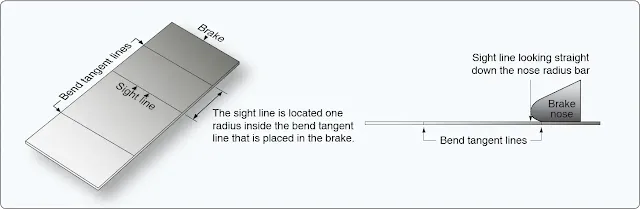Figure 9. Sight line

NOTE: A common mistake is to draw the sight line in the middle of the bend allowance area, instead of one radius away from the bend tangent line that is placed under the brake nose bar.

## Using a J-Chart To Calculate Total Developed Width

The J-chart, often found in the SRM, can be used to determine bend deduction or setback and the TDW of a flat pattern layout when the inside bend radius, bend angle, and material thickness are known. [Figure 10] While not as accurate as the traditional layout method, the J-chart provides sufficient information for most applications. The J-chart does not require difficult calculations or memorized formulas because the required information can be found in the repair drawing or can be measured with simple measuring tools.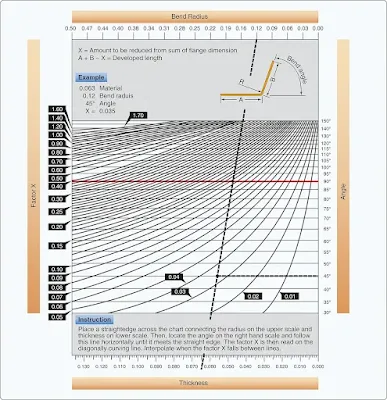Figure 10. J chart

When using the J-chart, it is helpful to know whether the angle is open (greater than 90°) or closed (less than 90°) because the lower half of the J-chart is for open angles and the upper half is for closed angles.

## How To Find the Total Developed Width Using a J-Chart

• Place a straightedge across the chart and connect the bend radius on the top scale with the material thickness on the bottom scale. [Figure 10]
• Locate the angle on the right hand scale and follow this line horizontally until it meets the straight edge.
• The factor X (bend deduction) is then read on the diagonally curving line.
• Interpolate when the X factor falls between lines.
• Add up the mold line dimensions and subtract the X factor to find the TDW.

Example 1

Material thickness = 0.063-inch
Bend angle = 90º
ML 1 = 2.00/ML 2 = 2.00

Use a straightedge to connect the bend radius (0.22-inch) at the top of the graph with the material thickness at the bottom (0.063-inch). Locate the 90° angle on the right hand scale and follow this line horizontally until it meets the straightedge. Follow the curved line to the left and find 0.17 at the left side. The X factor in the drawing is 0.17-inch. [Figure 11]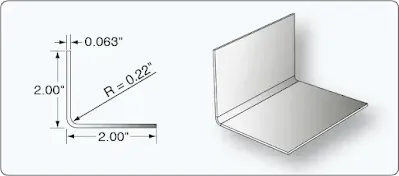Figure 11. Example 1 of J chart

Total developed width = (Mold line 1 + Mold line 2) – X factor
Total developed width = (2 + 2) – .17 = 3.83-inches

Example 2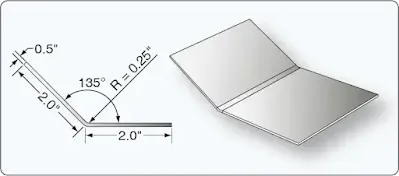Figure 12. Example 2 of J chart